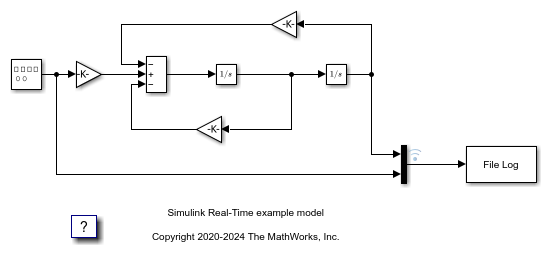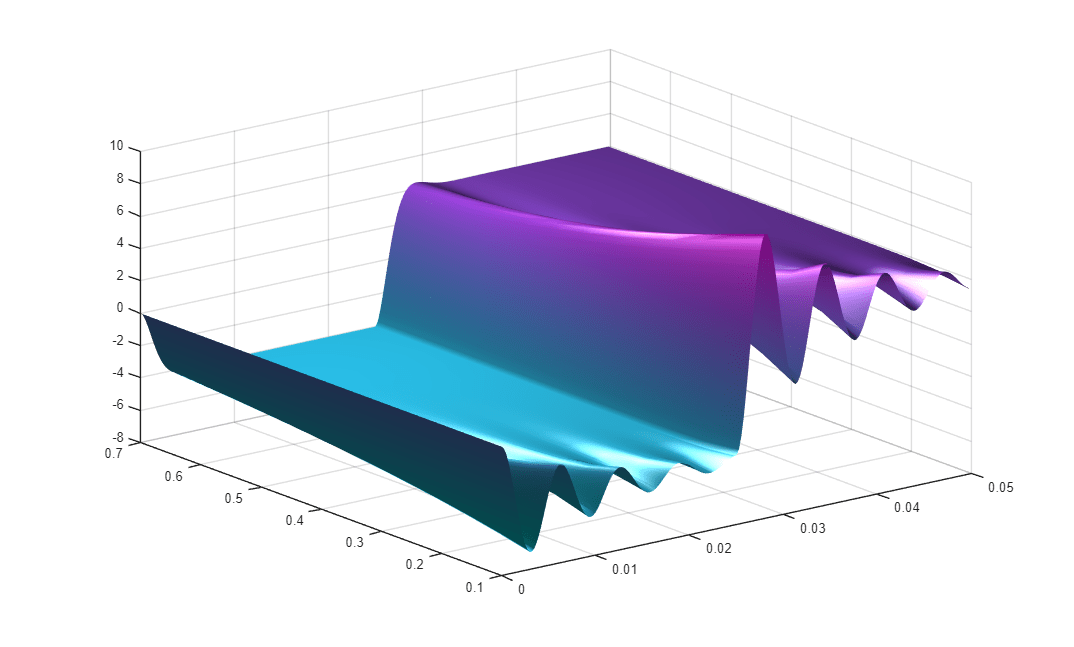# Parameter Tuning and Data Logging

This example shows how to use real-time parameter tuning and data logging with Simulink® Real-Time™. After the example builds the model and downloads the real-time application, `slrt_ex_param_tuning`, to the target computer, the example executes multiple runs with the gain 'Gain1/Gain' changed (tuned) before each run. The gain sweeps from 0.1 to 0.7 in steps of 0.05.

The example uses the data logging capabilities of Simulink Real-Time to capture signals of interest during each run. The logged signals are uploaded to the development computer and plotted. A 3-D plot of the oscillator output versus time versus gain is displayed.

Open the model, `slrt_ex_param_tuning`. The model configuration parameters select the `slrealtime.tlc` system target file as the code generation target. Building the model creates a real-time application, `slrt_ex_param_tuning.mldatx`, that runs on the target computer.

```model = 'slrt_ex_param_tuning'; open_system(model); ```Build the model and download the real-time application, `slrt_ex_param_tuning.mldatx`, to the target computer.

• Configure for a non-Verbose build.

```set_param(model,'RTWVerbose','off'); set_param(model,'StopTime','0.2'); evalc('slbuild(model)'); tg = slrealtime; load(tg,model); ```

### Run Model, Sweep 'Gain' Parameter, Plot Logged Data

Create the MATLAB® variable, `tg`, that contains the Simulink Real-Time target object. This object lets you communicate with and control the target computer.

• Create a Simulink Real-Time target object.

• Set stop time to 0.2s.

Task 2: Run the Model and Plot Results

Run the model, sweeping through and changing the gain (damping parameter) before each run. Plot the results for each run.

• If no plot figure exist, create the figure.

• If the plot figure exist, make it the current figure.

Task 3: Loop over damping factor z

• Set damping factor (Gain1/Gain).

• Start run of the real-time application.

• Store output data in `outp`, `y`, and `t` variables.

• Plot data for current run.

Task 4: Create 3-D Plot (Oscillator Output vs. Time vs. Gain)

• Loop over damping factor.

• Create a plot of oscillator output versus time versus gain.

• Create 3-D plot.

```figh = findobj('Name', 'parsweepdemo'); if isempty(figh) figh = figure; set(figh, 'Name', 'parsweepdemo', 'NumberTitle', 'off'); else figure(figh); end y = []; flag = 0; for z = 0.1 : 0.05 : 0.7 if isempty(find(get(0, 'Children') == figh, 1)) flag = 1; break; end load(tg,model); tg.setparam([model '/Gain1'],'Gain',2 * 1000 * z); tg.start('AutoImportFileLog',true, 'ExportToBaseWorkspace', true); pause(0.4); outp = logsOut{1}.Values; y = [y,outp.Data(:,1)]; t = outp.Time; plot(t,y); set(gca, 'XLim', [t(1), t(end)], 'YLim', [-10, 10]); title(['parsweepdemo: Damping Gain = ', num2str(z)]); xlabel('Time'); ylabel('Output'); drawnow; end if ~flag delete(gca); surf(t(1 : 200), 0.1 : 0.05 : 0.7, y(1 : 200, :)'); colormap cool shading interp h = light; set(h, 'Position', [0.0125, 0.6, 10], 'Style', 'local'); lighting gouraud title('parsweepdemo: finished'); xlabel('Time'); ylabel('Damping Gain'); zlabel('Output'); end ```### Close Model

When done, close the model.

```close_system(model,0); ```